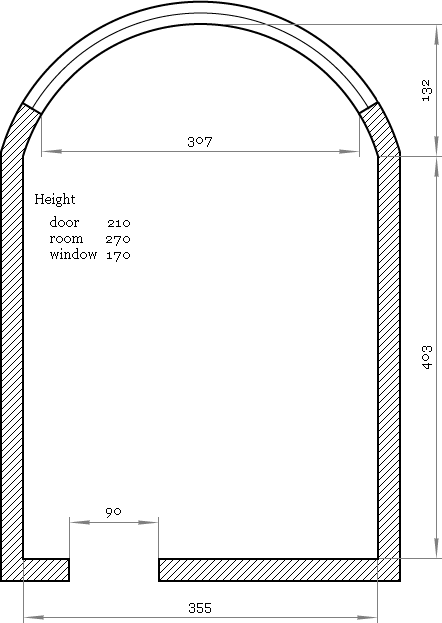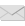The Art of Interface

# Librow scientific formula calculator — why and how

Category. Mathematical software development.

Abstract. Why and how Librow scientific formula calculator was created — philosophy and ideas behind, practical introduction to the project, script samples.## 1. Problem arise

Everything began from this...Fig. 1. Room layout.

I was to make renovation of my flat and first step to be done was to calculate how much of different things I needed. I measured everything, draw on paper and got a bunch of room plans like the one above. Plans looked simple, I had a handheld scientific calculator with expression evaluation support, so, the task looked easy.

First thing I discovered immediately it was not that easy to verify even simple formulas written in digits. It is much easier to check something like

``width × length``

than

``4.03 × 3.55``

Second discovery was the trend to recalculate the same values like square of the floor for different applications like calculation of amount of self-evening mixture and wooden flooring. So, here I ended up writing down some basic results on paper like listing of floor squares room by room.

The same happened with multistaged calculations like calculation of amount of tiling for bathroom, where surface tiling depends on bathtub location. So, third discovery was that calculator memory is not very convenient, because it is unnamed — it is good for abstract calculations where one to store some multiplier or coefficient but not real-world items like “part of the left wall covered with beige tiles”.

Fourth revelation came when the same thing was to be calculated for different rooms like amount of paint. Not to repeat the same job for different rooms I had written C++ application to calculate walls square. That was quite awkward: not to lose time for creation of file input or whatever I entered all input data hardcoded, commenting already calculated previous version and recompiling the code to run it for the next room.

And that was not the worst thing. The nastiest, fifth, thing came when ideas started to mix with reality: you had an idea to use 30×30 tiles for bathroom and 80 cm width bathtub but then went to shop to find tiles 30×40 look better and only tub of 70 cm width was available. So, the calculation job to be redone.

Add to this “one button at a time” calculator input and its two string display... Well, basic calculations in 21 century.

## 2. Conclusion

Out of my experience I got a wish list:

## I wish

• to write formulas in symbols or words, not in numbers;
• to store intermediate results under names, not under abstract “cell x”, “cell y”;
• to separate input data and formulas for their processing, so that the same succession of expressions could be run for different datasets;
• to save my job for future use in the case of changes;
• simple solution that allows easy modification of existing calculations.

## 3. Solution

Now, let me show how all that solved in Librow scientific calculator. So, first, here is our input data:

``````//   Room dimensions, m
3.55 −> mem[room width];
4.03 −> mem[room length];
2.7  −> mem[room height];
1.32 −> mem[bay depth];
.9  −> mem[door width];
2.1  −> mem[door height];
3.07 −> mem[window width];
1.7  −> mem[window height];``````

Enter data above into the calculator and save it as room.lcm file.

Clear the calculator window and enter paint data:

``````//   Paint consumption, Tikkurila Joker, l/m^2
1 /  8 −> mem[paint first cover];
1 / 11 −> mem[paint second cover];``````

Save the data as paint-joker.lcm file.

Now time for formulas. Here we are interested in squares for ceiling and walls. The room has rounded part, so some job should be done to get its square. As final step necessary amount of paint is calculated. So, clear the window and enter:

``````//   Bay radius
mem[room width]^2 / (8 * mem[bay depth]) + mem[bay depth] / 2 −> mem[bay radius];
//   Walls square: long walls + short wall + bay wall − window − door
(mem[room length] * 2 + mem[room width]
+ 2 * mem[bay radius] * arcsin(mem[room width] / (2 * mem[bay radius]))) * mem[room height]
− 2 * mem[bay radius] * arcsin(mem[window width] / (2 * mem[bay radius])) * mem[window height]
− mem[door width] * mem[door height] −> mem[square walls];
//   Ceiling square
mem[room length] * mem[room width]
− mem[room width] * (mem[bay radius] − mem[bay depth]) / 2 −> mem[square ceiling];
//   Amount of paint for walls
mem[square walls] * (mem[paint first cover] + mem[paint second cover]);
//   Amount of paint for ceiling
mem[square ceiling] * (mem[paint first cover] + mem[paint second cover]);``````

Save the script as square-bay.lcl file.

Now click Memory >> Load and load into memory previously saved room.lcm file. You can see the loaded data in the Memory window. Repeat the same for paint-joker.lcm file — paint data will be added to memory. Now hit Ctrl+Shift+= to evaluate squares and amount of paint.

## 4. Discussion

As you can see, Librow scientific calculator allows easy separation of datasets and calculation logic — so that the same calculation script could be run for different inputdata. In my case it allows making evaluations for different rooms and check out different paints. For instance, to see another paint consumption it is enough just load another memory file:

``````//   Paint consumption, Tikkurila Luja, l/m^2
1 / 5 −> mem[paint first cover];
1 / 8 −> mem[paint second cover];``````
As well one can easily modify the calculation script for rectangular rooms, add another window or door, add calculation of flooring, etc. — expressions for all that are already there. For example, here is the script for rectangular room, got from the script above by removing expressions for bay squares:

``````//   Walls square: walls − window − door
(mem[room length] + mem[room width]) * 2 * mem[room height]
− mem[window width] * mem[window height]
− mem[door width] * mem[door height] −> mem[square walls];
//   Ceiling square
mem[room length] * mem[room width] −> mem[square ceiling];
//   Amount of paint for walls
mem[square walls] * (mem[paint first cover] + mem[paint second cover]);
//   Amount of paint for ceiling
mem[square ceiling] * (mem[paint first cover] + mem[paint second cover]);``````

And the calculator provides effective memory management, so that one is not lost among memory cells.

To be exact the data above and scripts themselves are my real tools I have written as soon as I have created Librow scientific calculator. You can copy the scripts from the screen or download them here:

## 5. About Librow professional scientific formula calculator

Librow professional formula calculator is an advanced version of the Librow free scientific formula calculator, so, everything said above is true for it as well. The difference between them is professional version has some extra:

## Librow professional fomula calculator

• supports complex numbers
• supports special functions:
• can evaluate sum and product of series
• can generate random numbers

The features could be of help in advanced scientific calculations.Write to the author of the article — Sergey Chernenko